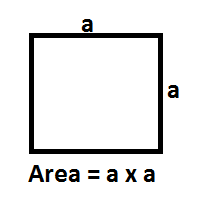# Area of a Square Formula

In geometry, a Square is a flat figure or shape which has 4 equal sides and every angle is a right angle i.e. 90° and it shows equal sides. From this, it follows that the opposite sides are also parallel. It is also described as a rectangle which has 2 adjacent sides have equal length.  The area of a square found out by the amount of space occupied within the square. Below mentioned is a formula to calculate the area of square.  It is best known of the quadrilaterals in geometry. Square is simply a specific case of a regular polygon, in this case with 4 equal sides. All the facts and properties described for regular polygons apply to the square.AreoSquarFormula2
Where,
a is the length of the side of a square

### Solved Examples

Question 1: Find the area of the square of side 16 cm?
Solution:

Side of the square = a = 16 cm
Area of the square
= a2
= 162 cm2
= 256 cm2
Question 2: Find the length of the square whose area is 529 cm2?
Solution:
Area of the square = A = 529 cm2
Side of the square = a = ?

Area of the square = A = a2
⇒ 529 cm= a
⇒ a = 529
⇒ a = 23 cm
Hence the length of the side of this square is 23 cm.